Courses

Test: Strength of Solutions

10 Questions MCQ Test Chemistry for JEE | Test: Strength of Solutions

Description
Attempt Test: Strength of Solutions | 10 questions in 15 minutes | Mock test for JEE preparation | Free important questions MCQ to study Chemistry for JEE for JEE Exam | Download free PDF with solutions
QUESTION: 1

Molality is expressed in

Solution:

Molality = moles of solute / kg of solvent.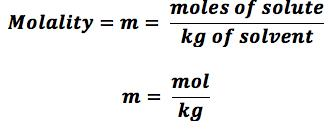QUESTION: 2

The level of contamination of chloroform was found to be 15 ppm. It means 15 g of chloroform is present in how many grams of solution?

Solution:

1 ppm is equivalent to 1 part out of 1 million (106) parts.
∴ Mass percent of 15 ppm chloroform in water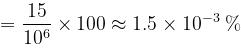⇒ 1.5 x 10-3 g chloroform present in 100 g water
Thus, 15g chloroform will be present in water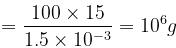QUESTION: 3

Mole fraction of glycerine, C3H5(OH)3 in a solution containing 36 gm of water and 46 gm of glycerine is:

Solution:

Molecular mass of glycerine = 92
Molecular mass of water = 18

► No. of moles of glycerine = 46/92 = 0.5 moles
► No. of moles of water = 36/18 = 2 moles

Thus, Mole fraction of glycerine = No. of moles of glycerine/(No. of moles of glycerine + No. of moles of water) = 0.5/(2 + 0.5)  = 0.20

QUESTION: 4

Calculate the molality of 12.5% w/w sulphuric acid?

Solution:

12.5% w/w means 12.5 g in 100 g of solution.

► Weight of solvent = 100 g – 12.5 g = 87.5 g.
► Number of moles of sulphuric acid = 12.5/ 98 = 0.127 mol

⇒ molality = 0.127 x 1000/87.5 = 1.45 m

QUESTION: 5

When the solvent is in solid state, solution is

Solution:

When both the solute and solvent are in solid state, then the solution is called as solid solution or solid sol.

QUESTION: 6

The number of moles of KCl in 3 L of 3 M solution is

Solution:

In 3M there will be 3 moles per litre therefore in 3 litres there will be 9 moles.

QUESTION: 7

A sample of 300.0 g of drinking water is found to contain 38 mg Pb. What this concentration in parts per million?

Solution:

We use parts per million to express the concentrations of solutions that contain very, very small amounts, often called trace amounts, of a given solute.

More specifically, a solution's concentration in parts per millions tells you the number of parts of solute present for every

10= 1,000,000

parts of solution. You can thus say that a 1 ppm solution will contain exactly 1 g of solute for every 106g of solution.

In your case, you know that you have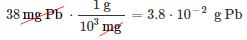in exactly
300.0 g = 3.000 ⋅ 102g solution

This means that you can use this known composition as a conversion factor to scale up the mass of the solution to 106g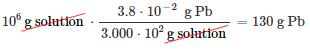Since this represents the mass of lead present in exactly 106g of solution, you can say that the solution has a concentration of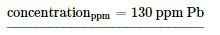QUESTION: 8

What is the mass of NaCl required to prepare 0.5 liters of a 2.5 molar solution of NaCl?

Solution:

The molecular weight of NaCl is 58.44 g/mol

So, one mole of NaCl weighs 58.44 g.

A 2.5 M solution is 2.5 moles per liter (Molarity is just the number of moles per liter).

Therefore, 0.5 L would contain 1.25 mol. Hence, you would need 1.25 × 58.44 g = 73 g.

QUESTION: 9

The molarity of a solution obtained by mixing 750 ml of 0.5(M) HCl with 250ml of 2(M) HCl will be?

Solution:

The molarity of a resulting solution is given by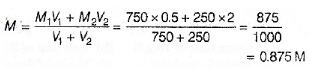QUESTION: 10

Mole fraction of ethyl chloride and methanol in a ternary solution is 0.6 and 0.32 respectively. What is the mole fraction of third component. Also identify the solvent in this ternary solution.

Solution:

Mole fraction of third component is 1 - (0.6 + 0.32) = 0.08.
Since ethyl chloride has highest mole fraction so it is the solvent.Use Code STAYHOME200 and get INR 200 additional OFF Use Coupon Code

Track your progress, build streaks, highlight & save important lessons and more!

Similar ContentRelated tests